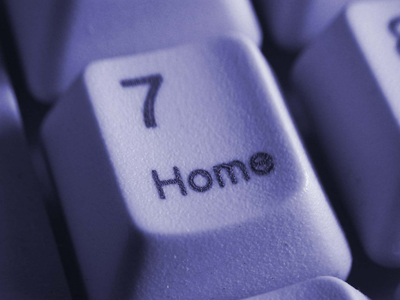The square root of 49 can be 7 or -7.

# Level 3-4 Algebra - Square Roots

So, now you've become familiar with powers and square numbers. But there is another associated term which you'll come across a great deal in KS3 Maths - square roots.

No, they're not parts of plants shaped like cubes! Square roots are the opposite of square numbers. Here are a couple of examples: the square of 3 is 9 (3 x 3) so the square root of 9 is 3. The square of 8 is 64 (8 x 8) so the square root of 64 is 8. All numbers have their own square root. But most are not as easy to work out as the above examples. The square root of 4 is 2 and the square root of 9 is 3. But what is the square root of 6? The answer is 2.449489742783178. Sometimes you just have to use a calculator!

1.
What is the square root of 100?
2 or -2
10 or -10
25 or -15
50 or -50
Minus numbers multiplied by themselves always give a positive answer. So, strange as it seems, -10 x -10 = 100
2.
What is the square root of 81?
3 or -3
6 or -6
9 or -9
12 or -12
9 x 9 = 81 AND -9 x -9 = 81
3.
You are given the two numbers '9 squared' and '81'. How do they relate to each other?
81 is the larger number
9 squared is the larger number
They are both the same
They are in no way related
9 x 9 = 81
4.
What is the quickest way to find the square root of 8?
Divide 8 by 2
Multiply 8 x 2
Take 2 from 8
Use a calculator
Some square roots are not whole numbers and can be very difficult to work out. The square root of 8 is approximately 2.828427. We used a calculator to find out!
5.
The √ sign is referred to as what?
Square
Squared
Square root
Square rooted
It's important you get to know what the square root sign looks like
6.
Which of these numbers has a whole number as a square root?
30
32
34
36
6 x 6 = 36
7.
Which of these figures does not have a whole number as a square root?
25
35
49
64
The square root of 35 is 5.916079783099616
8.
You are given the two numbers '3 squared' and 'the square root of 100'. How do they relate to each other?
3 squared is the larger number
The square root of 100 is the larger number
They are both the same
There is no way of telling
The square root of 100 could be either 10 or -10 and we have no way of knowing which square root the question requires!
9.
What is the square of 9?
3
6
9
81
Did you spot that we asked for the 'square' and NOT the 'square root'?
10.
What is the square root of 16?
2 or -2
4 or -4
6 or -6
8 or -8
Both 4 x 4 and -4 x -4 = 16